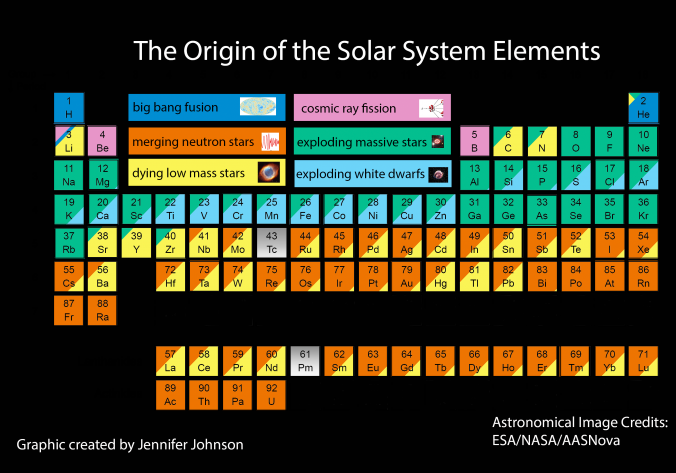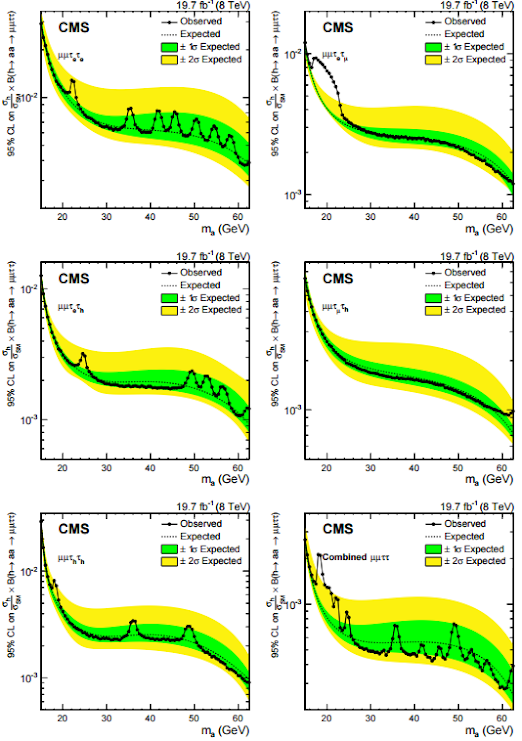## Tuesday, January 10, 2017 ...//### CMS: a small Higgs to $$\mu\mu\tau\tau$$ decay hint of a $$19\GeV$$ boson

Statistics hasn't ceased to hold in 2017, even though the latter is a prime integer. So excesses keep on appearing in the LHC experiment, including the newly published CMS preprint about an analysis based on 20 inverse femtobarns of the 2012, i.e. center-of-mass energy $$8\TeV$$ data:

Search for light bosons in decays of the $$125\GeV$$ Higgs boson in proton-proton collisions at $$\sqrt{s} = 8\TeV$$ (by Aaallah and 2000+ co-authors)
They only look at events in which the Higgs boson discovered in 2012 is produced – the number of collisions of this type (which were not known at all before late 2011) is so high that the experimenters may look at small special subsets and still say something interesting about these subsets.Off-topic but fun chart of the day. Source.

So they focus on events in which the $$125\GeV$$ Higgs decays to four fermions, as if it were first decaying to two lighter bosons, $$h\to aa$$. The final states they probe include "four taus", "two muons plus two taus", and "two muons and two bottom quarks". It's not quite clear to me why they omit the other combinations, e.g. "two taus and two bottom quarks" etc. (except that I know that "four muons" was focused on in a special paper), but there may be some mysterious explanation.

They say that there's no statistical excess anywhere. But what this statement means should be interpreted a bit carefully because it potentially understates the deviations from the Standard Model they are seeing. By "no statistical excesses", they mean that there's no excess whose global significance, i.e. significance reduced by the look-elsewhere correction, exceeds 2 sigma.

In other words, the statement "nothing can be seen here" is compatible with the existence of more than 2-sigma – and perhaps a bit higher – excesses if evaluated locally, i.e. without any look-elsewhere reduction of the confidence level. And yes, those are seen.Click to zoom in.

This chart – Figure 6 on Page 18 (page 20 of 48 according to the PDF file) – shows the Brazil bands for the final state with $$\mu\mu\tau\tau$$. The tau leptons quickly decay and they split the final channels according to the decay products of these $$\tau$$ as well – although, even in this case, it doesn't quite seem to me that they have listed all the options. ;-)

You see that the black, observed curves are sometimes smooth, sometimes very wiggly. The wiggles are sometimes unusually periodic – like in the upper left channel. But the most remarkable excess is seen in the upper right channel in which the two $$\tau$$ leptons decay to one electron and one muon, respectively (plus neutrinos – missing energy).

You see that the distance from the Brazil band – for the mass $$m_a$$ of the new light bosons depicted on the $$x$$-axis that is around $$20\GeV$$ – is substantial. If a Brazilian soccer player deviated from the Brazilian land this severely, he would surely get drowned in the Atlantic Ocean. It looks like a "many sigma" deviation locally and I am a bit surprised that it doesn't make it to 2 sigma globally.

Four other channels show nothing interesting around $$m_a\sim 20\GeV$$ but the last one, the lower left channel with both $$\tau$$ decaying hadronically – shows a small local (and in this case, much narrower – the energy is measured accurately because no energy is lost to ghostly neutrinos in hadronic decays) excess for $$m_a\sim 19\GeV$$. When these two excesses (and the flat graphs from the other channels) are added, we see the combined graph in the lower right corner which shows something like a locally 3-sigma excess for $$m_a\sim 19\GeV$$.

It's almost certainly a fluctuation. If it weren't one, it should be interpreted as the "second Higgs boson" in a general 2HDM (two-Higgs-doublet model) which is ugly and unmotivated by itself. But such models may be typically represented as the Higgs part of the NMSSM (next-to-minimal supersymmetric standard model) which is very nice and explains the hierarchy problem more satisfactorily than MSSM. Even though it also has two Higgs doublets and therefore two CP-even neutral Higgs bosons in them, MSSM itself cannot reproduce these rather general 2HDM models.

It would be of course exciting if the LHC could suddenly discover a new $$20\GeV$$ Higgs-like boson and potentially open the gates to truly new physics like supersymmetry but like in so many cases, I would bet on "probably not" when it comes to this modest excess.

Don't you find it a bit surprising that now, in early 2017, we are still getting preprints based on the evaluation of the 2012 LHC data? The year was called "now" some five years ago. Are they hiding something? And when they complete an analysis like that, why don't they directly publish the same analysis including all the 2015+2016 = 4031 data as well? Surely the analysis of the same channel applied to the newer, $$13\TeV$$ data is basically the same work.

Maybe they're trying to pretend that they're writing more papers, and therefore doing more work? I don't buy it and neither should the sponsors and others. Things that may be done efficiently should be done efficiently. If it leads to the people's having more time to enjoy their lives instead of writing very similar long papers that almost no one reads, they should have more time to enjoy their life – and to collect energy needed to make their work better and more happily.

Another new CMS paper searching for SUSY with top tagging shows no excess, not even 2-sigma excess locally, but there's a nice more than 1-sigma repulsion from the point with a $$600\GeV$$ top squark and a $$300\GeV$$ neutralino or so.#### snail feedback (0) :

(function(i,s,o,g,r,a,m){i['GoogleAnalyticsObject']=r;i[r]=i[r]||function(){ (i[r].q=i[r].q||[]).push(arguments)},i[r].l=1*new Date();a=s.createElement(o), m=s.getElementsByTagName(o);a.async=1;a.src=g;m.parentNode.insertBefore(a,m) })(window,document,'script','//www.google-analytics.com/analytics.js','ga'); ga('create', 'UA-1828728-1', 'auto'); ga('send', 'pageview');